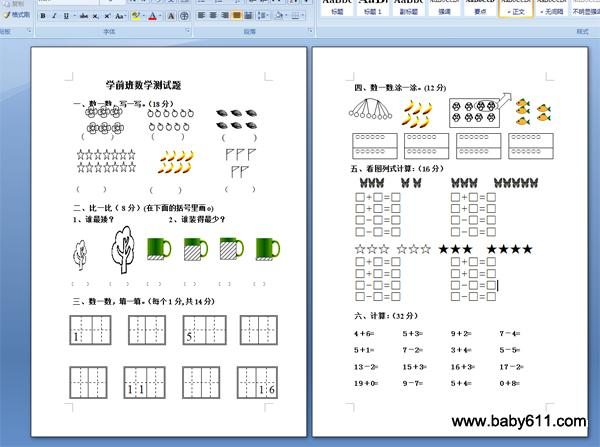# 春学期幼儿园学前班数学测试题一、数一数，写一写。（18分）

（      ）       （      ）        (       )

（      ）           (       )           （      ）

二、比一比（8分）(在下面的括号里画o)

1、谁最矮？               2、谁装得最少？

（     ）        （      ）       （    ）         （   ）         （   ）         （   ）

三、数一数，填一填。（每个1分,共14分)

15

1116

四、数一数.涂一涂。(12分)

五、看图列式计算：（16分）

□+□=□        □+□=□

□+□=□        □+□=□

□-□=□        □-□=□

□-□=□        □-□=□

☆☆☆ ☆☆☆ ★★★  ★★★★

□+□=□        □+□=□

□+□=□        □+□=□

□-□=□        □-□=□

□-□=□        □-□=□

六、计算：（32分）

4＋6=5＋3=9＋2=7－4=

5＋1=7－2=3＋4=5－5=

13－2=15＋3=16＋3=17－2=

19＋0=9－7=5＋4=0＋8=

• 幼儿园试卷分类
• 将此试卷分享到：
• 学前班试卷最新更新